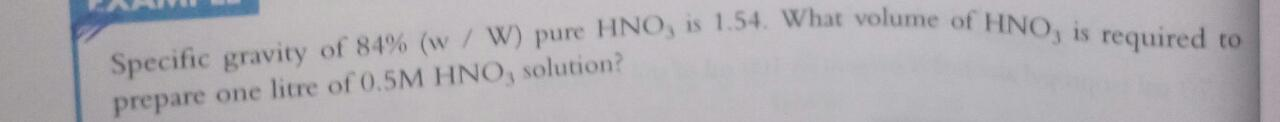# the hno3 is 84% pure with specific gravity 1.54 volume of hno3 needed to make 0.5M solution in 1litre solution​5 years ago
Dear student

molecular weight of nitric acid = 63g
Specific Gravity  = 1.54
% purity = 84%
number of replacable hydrogens = 1
Gram Equivalent Weight =molecular weight/replaceable hydrogens
63/1 = 63g

Required substance weight = eq. wt. x Normality x Volume (in L)
= 63gm x 0.5N X 1 Litres = 31.5 g   [here Normality =molarity, as no.of H+ ions =1]
Dilution Factor = Specific Gravity x concentration of pure acid.
=1.54 x 0.84 = 1.3gm

Volume required = mass required/dilution factor
= 31.5/1.3 = 24.2 ml
Regards